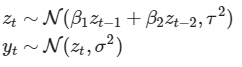# Using lax.scan for time series in NumPyro

Hi! I recently started using numpyro for time series models and am having some problems in using `lax.scan` functionalities.

In particular, here I am trying to implement a simple state-space model with order-2 autoregressive dynamics, something like:.

A vanilla (i.e. without `lax.scan`) implementation would look something like the following:

``````def model(T, T_forecast, obs=None):
beta1 = numpyro.sample(name="beta_1", fn=dist.Normal(loc=0., scale=1))
beta2 = numpyro.sample(name="beta_2", fn=dist.Normal(loc=0., scale=1))
tau = numpyro.sample(name="tau", fn=dist.HalfCauchy(scale=1))
sigma = numpyro.sample(name="sigma", fn=dist.HalfCauchy(scale=1))
z_prev1 = numpyro.sample(name="z_1", fn=dist.Normal(loc=0, scale=1))
z_prev2 = numpyro.sample(name="z_2", fn=dist.Normal(loc=0, scale=1))

Z = []
Y = []
for t in range(2, T):
z_t_mean = beta1*z_prev1 + beta2*z_prev2
z_t = numpyro.sample(name="z_%d"%(t+1), fn=dist.Normal(loc=z_t_mean, scale=tau))
Z.append(z_t)
z_prev1 = z_prev2
z_prev2 = z_t

for t in range(T, T+T_forecast):
z_t_mean = beta1*z_prev1 + beta2*z_prev2
z_t = numpyro.sample(name="z_%d"%(t+1), fn=dist.Normal(loc=z_t_mean, scale=tau))
Z.append(z_t)
z_prev1 = z_prev2
z_prev2 = z_t

numpyro.sample(name="y_obs", fn=dist.Normal(loc=np.array(Z[:T-2]), scale=sigma), obs=obs[:T-2])
numpyro.sample(name="y_pred", fn=dist.Normal(loc=np.array(Z[T-2:]), scale=sigma), obs=None)
return Z
``````

where I am trying to be Bayesian over all model parameters (and predictions). This implementation works fine, however, because of the `for`-loops, this simple model takes way too much time to compile… Following the time-series tutorial on the numpyro documentation I came up with what would seem to me as an equivalent implementation (this time using `lax.scan`):

``````def scan_fn(carry, x):
beta1, beta2, z_prev1, z_prev2 = carry
z_t = beta1*z_prev1 + beta2*z_prev2
z_prev1 = z_prev2
z_prev2 = z_t
return (beta1, beta2, z_prev1, z_prev2), z_t

def model(T, T_forecast, obs=None):
beta1 = numpyro.sample(name="beta_1", fn=dist.Normal(loc=0., scale=1))
beta2 = numpyro.sample(name="beta_2", fn=dist.Normal(loc=0., scale=1))
tau = numpyro.sample(name="tau", fn=dist.HalfCauchy(scale=1))
sigma = numpyro.sample(name="sigma", fn=dist.HalfCauchy(scale=1))
z_prev1 = numpyro.sample(name="z_1", fn=dist.Normal(loc=0, scale=1))
z_prev2 = numpyro.sample(name="z_2", fn=dist.Normal(loc=0, scale=1))

_Z_exp = [z_prev1, z_prev2]
__, zs_exp = lax.scan(scan_fn, (beta1, beta2, z_prev1, z_prev2), None, T+T_forecast-2)
Z_exp = jnp.concatenate((jnp.array(_Z_exp), zs_exp), axis=0)

Z = numpyro.sample(name="Z", fn=dist.Normal(loc=Z_exp, scale=tau))
numpyro.sample(name="y_obs", fn=dist.Normal(loc=Z[:T], scale=sigma), obs=obs[:T])
numpyro.sample(name="y_pred", fn=dist.Normal(loc=Z[T:], scale=sigma), obs=None)
return Z_exp
``````

where I use `lax.scan` to collect the expected values for the latent state `z_t`. The problem is that when I try running this model, MCMC inference does not converge to meaningful values… Am I missing something evident on how the `lax.scan` function works or should I implement the model differently?

Thanks!

You can have a look at this code to add noise (parameter tau) at each timestep

2 Likes

@Daniele Have you tested if your `lax.scan` version and `for` loop version gives the same result?

1 Like

@vincentbt thanks for the suggestion. I actually tried adding noise at each time step and did not mention it here (mostly because results did not change much) with the following implementation (which seems quite similar to the one in the post you are mentioning):

``````def scan_fn(carry, tau_t):
beta1, beta2, z_prev1, z_prev2 = carry
z_t = beta1*z_prev1 + beta2*z_prev2 + tau_t
z_prev1 = z_prev2
z_prev2 = z_t
return (beta1, beta2, z_prev1, z_prev2), z_t

def model(T, T_forecast, obs=None):
beta1 = numpyro.sample(name="beta_1", fn=dist.Normal(loc=0., scale=1))
beta2 = numpyro.sample(name="beta_2", fn=dist.Normal(loc=0., scale=1))
tau = numpyro.sample(name="tau", fn=dist.HalfCauchy(scale=jnp.ones(T+T_forecast)*))
sigma = numpyro.sample(name="sigma", fn=dist.HalfCauchy(scale=1))
z_prev1 = numpyro.sample(name="z_1", fn=dist.Normal(loc=0, scale=1))
z_prev2 = numpyro.sample(name="z_2", fn=dist.Normal(loc=0, scale=1))

_Z_exp = [z_prev1 + tau, z_prev2 + tau]
__, zs_exp = lax.scan(scan_fn, (beta1, beta2, z_prev1, z_prev2), tau[2:], T+T_forecast-2)
Z_exp = jnp.concatenate((jnp.array(_Z_exp), zs_exp))

numpyro.sample(name="y_obs", fn=dist.Normal(loc=Z_exp[:T], scale=sigma), obs=obs[:T])
numpyro.sample(name="y_pred", fn=dist.Normal(loc=Z_exp[T:], scale=sigma), obs=None)
return Z_exp
``````

but I will definitely take a better look at the post@fehiepsi Yes I did try them both and the `for`-loop version manages to model the data sufficiently well (as well as imputing missing values) while the `lax.scan` version gives meaningless results (although sampling seems to have converged)… does this answer your question?

@Daniele I meant a test for the output of `lax.scan` and `for` loop givens the same inputs.

Looking at two models again, it seems to me that they are different. In the first model, `z_prev2 = z_t`, which is a noisy sample of `z_t_mean`, while in the second model, `z_prev2 = z_t_mean`. To make them equivalent (up to reparamterization), I think you can sample the noise first:

``````noises = numpyro.sample("noises", fn=dist.Normal(0, 1), sample_shape=(T,))
``````

then in `lax.scan`, you can convert the statement

``````z_t = numpyro.sample(name="z_%d"%(t+1), fn=dist.Normal(loc=z_t_mean, scale=tau))
``````

to something like

``````z_t_mean = beta1*z_prev1 + beta2*z_prev2
z_t = z_t_mean + tau * noise
``````

This is what @vincentbt suggested I believe.

1 Like

@fehiepsi In that case yes, I did check and the output is indeed equivalent.

I also implemented the model as you suggested and it indeed starts working better. However, the MCMC chains seem to achieve a lower number of effective samples compared to the “vanilla” implementation (therefore requiring some tuning of priors/step size/num_steps). Could there be a specific reason for this? And if the reason is indeed reparametrization, are there any “best practices” for making the estimation more stable?

Thanks